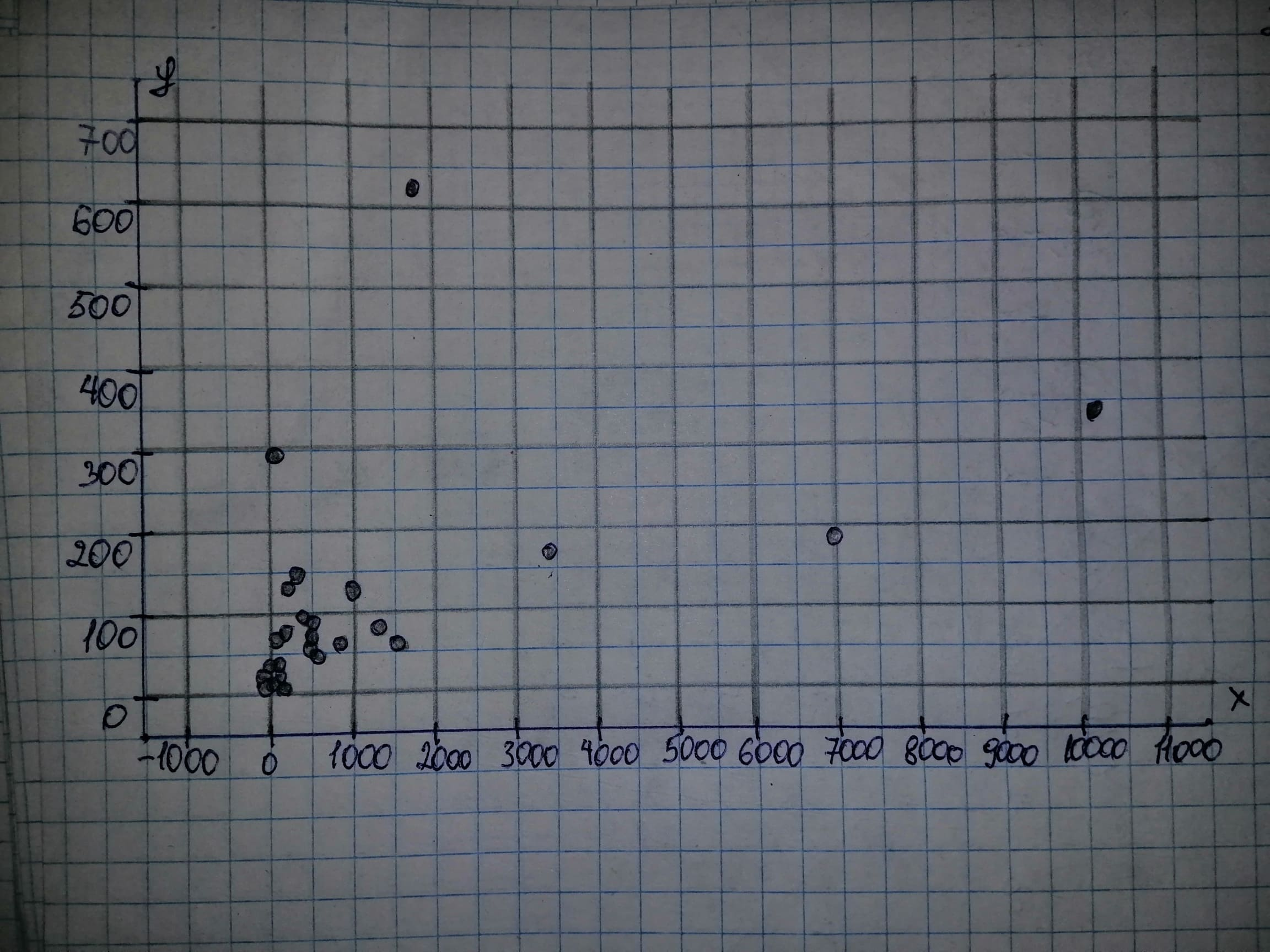mattgondek4

2020-10-21

An issue of BARRON’S presented information on top wealth managers in the United States, based on individual clients with accounts of \$1 million or more. Data were given for various variables, two of which were number of private client managers and private client assets. a) Obtain a scatterplot for the data. b) Decide whether finding a regression line for the data is reasonable. If so, then also do parts (c)–(f). c) Determine and interpret the regression equation for the data. d) Identify potential outliers and influential observations. e) In case a potential outlier is present, remove it and discuss the effect. f) In case a potential influential observation is present, remove it and discuss the effect.ottcomn

Expert

Given: a) Managers is on the horizontal axis and Assets is on the vertical axis.b) It is reasonable to find a regression lien for the data if there is no strong curvature present in the scatterplot. We note that there is no strong curvature in the scatterplot of part (a) and thus it is reasonable to find a regression line for the data. c) Let us first determine the necessary sums:

Next, we can determine

The estimate b of the slope :
The mean is the sum of all values divided by the number of values:
The estimate a of the intercept $\alpha$ is the average of y decreased by the product of the estimate of the slope and the average of x. General least-squares equation: in the general least-squares equation: d) The right most point appears to be an influential outlier, because it lies extremely close to the regression line and lies far from the other points in the data set. The point at the top of the scatterplot appears to be an outlier, because it lies far from the data as well. There appears to be a few other outliers as well, but we will only use these two points in parts (e) and (f). e) Let us first determine the necessary sums:

Next, we can determine

Do you have a similar question?